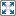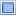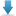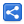# Java Regex - Lookahead Assertions

[Last Updated: Dec 6, 2018]

Lookaheads are zero length assertions, that means they are not included in the match.

They only assert whether immediate portion ahead of a given input string's current portion is suitable for a match or not.

Lookbehind is another zero length assertion which we will cover in the next tutorial. Generally, both assertions are known as Lookaround assertions.

All lookaround are non-capturing.

General syntax for a lookahead: it starts with a parentheses `(?` followed by another meta-character (either `=` or `!`) followed by assertion regex (all regex expressions are allowed here) followed by closing parentheses `)`.

There are two kind of lookahead assertions: Positive Lookahead and Negative Lookahead, and each one of them has two syntax : 'assertion after the match' and 'assertion before the match'. Both of them apply the assertion condition ahead of their position. Let's start exploring each with examples.

Positive lookahead is usually useful if we want to match something followed by something else.
Syntax: `(?=X)`

For example [a-z](?=[0-9])

Match a character followed by a digit.

How engine works?

1. First the character at the current position in the input string is attempted for a match, which should satisfy the first part of the expression, '[a-z]'
2. If the character has matched then engine will read the assertion part '(?=[0-9])'.
3. If the next character in the input string satisfies the assertion part (i.e. if the character is a digit) then we have a match otherwise the engine will reject the current character and move to the next position to repeat the process.
4. During reading the assertion part, engine doesn't actually consume any input string for the assertion part. That is it doesn't effect the position given by `Matcher#start()` (in case if we have any match anywhere after assertion part).

Note that the engine remembers the match only within the assertion block i.e. within (?=[0-9]) in above example. It gives up the match as soon as it exists the block, only returning: match or no match.

```/* Find all words ending with comma. (Not using lookahead yet.)*/
Pattern.compile("[a-z]+,")       .matcher("bat, cat, dog, fox")       .find();//matches:  'bat,' at 0-4, 'cat,' at 5-9, 'dog,' at 10-14
//'bat, cat, dog, fox'

/* Use positive lookahead, finding all words ending with comma without including comma in the match so we don't have to remove them manually.*/
Pattern.compile("[a-z]+(?=,)")       .matcher("bat, cat, dog, fox")       .find();//matches:  'bat' at 0-3, 'cat' at 5-8, 'dog' at 10-13
//'bat, cat, dog, fox'

Pattern.compile("[a-z](?=[0-9])")       .matcher("a7 bb")       .find();//matches:  'a' at 0-1
//'a7 bb'

/* Digits followed by a space followed by cm.*/
Pattern.compile("\\d+(?=\\scm)")       .matcher("200 cm")       .find();//matches:  '200' at 0-3
//'200 cm'

/* Finding all words followed by 's' using positive lookahead*/
Pattern.compile("[a-z]+(?=s)")       .matcher("ages mix uses woes")       .find();//matches:  'age' at 0-3, 'use' at 9-12, 'woe' at 14-17
//'ages mix uses woes'

/* In above example, since '+' is greedy quantifier, it matches 'use' as a complete match. Let's change it to reluctant and see the difference. Now it will match 'u' as a separate match (reluctant quantifiers yield shortest matches) because it is followed by 's' too.*/
Pattern.compile("[a-z]+?(?=s)")       .matcher("ages mix uses woes")       .find();//matches:  'age' at 0-3, 'u' at 9-10, 'se' at 10-12,                //'woe' at 14-17
//'ages mix uses woes'

/* Finding all characters followed by i */
Pattern.compile("[a-z](?=i)")       .matcher("bit car biz ice")       .find();//matches:  'b' at 0-1, 'b' at 8-9
//'bit car biz ice'

/* Let's replace [a-z] class with . (a dot) which means any character. Now white-space will also be included in the match.*/
Pattern.compile(".(?=i)")       .matcher("bit cat biz ice")       .find();//matches:  'b' at 0-1, 'b' at 8-9, ' ' at 11-12
//'bit cat biz ice'

/* Finding the character followed by a white-space.*/
Pattern.compile("[a-z](?=\\s)")       .matcher("bit cat biz ice")       .find();//matches:  't' at 2-3, 't' at 6-7, 'z' at 10-11
//'bit cat biz ice'

/* Using alteration in the lookahead part. We can use all valid constructs inside assertion part.*/
Pattern.compile("[a-z](?=[0-9]|[a-d])")       .matcher("a10 c2 a1 ez dd")       .find();//matches:  'a' at 0-1, 'c' at 4-5, 'a' at 7-8, 'd' at 13-14
//'a10 c2 a1 ez dd'

Pattern.compile("[a-z](?=1)")       .matcher("a10 a1 1 a a")       .find();//matches:  'a' at 0-1, 'a' at 4-5
//'a10 a1 1 a a'

Pattern.compile("[a-z](?=1\\s)")       .matcher("a10 a1 1 a a")       .find();//matches:  'a' at 4-5
//'a10 a1 1 a a'

```

Rewriting above example: (?=[a-z][0-9])[a-z].

How engines works?

1. At a given position of the input string, the engine will read the lookahead part '(?=[a-z][0-9])' first.
2. For the first part [a-z]: if at the current position of the input string there is a character between a to z then good.
For Second part [0-9]: if there's a digit at the following position then the current position satisfies the entire lookahead part otherwise it does not. During this time no input sequence is consumed.
3. If current input character does not satisfy the lookahead part, the engine will reject the current character as a match and will move on to the next character
4. If current input position satisfies the lookahead part then engine will actually apply the match at the current position. The next part of the expression (just after the assertion part) is [a-z]. As we know engine discards all matches of the assertion as soon as it's out of the assertion part (last section discussion) it will match the current input string with the the next '[a-z]' regex part normally. It just like engine doesn't have any memory of the last assertion. The assertion only helped the engine to decided whether the current position should be attempted for a match or not. If no then engine would have moved to next position already.
In our example if at current position we have a character matching '[a-z]' (of course we must have it, that's why assertion passed) then we have a match.

Note that this pattern achieves the same result as last section above ("Positive Lookahead before the Match"), but it is less efficient because [a-z] is read twice. We cannot avoid using them in some scenarios. Also not always using two variants will give the same results (as you will see some examples in the right column).

##### Part repetitions

In above example note that in (?=[a-z][0-9])[a-z], we repeated [a-z] twice, one inside the assertion part and one outside. The reason is: whatever we have for the engine to assert, we have to have a match for it as well. We only repeat the part outside which we are interested to have match with. If we don't have it, there won't be any runtime exception but there will be no match either (the assertion will fail itself).
Also we can have subset of whatever we have in the assertion part. Or we can have that as more/less restrictive than assertion part.
For example
Expression: (?=\\w[0-9])7
input string: 76
This will have a match of '7' because during assertion checking \\w matches '7' and [0-9] matches '6'.
In fact the expression '(?=\\w)7' will also match input string '7' (there's not that typical 'followed by something' concept though)
Please see examples in right columns, specially the one with input string 'brotherly isothermal'.

```Pattern.compile("(?=[a-z][0-9])[a-z]")       .matcher("a7 bb")       .find();//matches:  'a' at 0-1
//'a7 bb'

/* Our lookahead is: a character followed by a digit followed by a character: but we are only want to have match for first character.*/
Pattern.compile("(?=[a-z][0-9][a-z])[a-z]")       .matcher("a7a bbb 9z9")       .find();//matches:  'a' at 0-1
//'a7a bbb 9z9'

Pattern.compile("(?=[a-z][0-9])[0-9]")       .matcher("7a bc d8 9w")       .find();//no matches

/* This works.*/
Pattern.compile("(?=[a-z][0-9])d[0-9]")       .matcher("7a bc c6 d8 9w")       .find();//matches:  'd8' at 9-11
//'7a bc c6 d8 9w'

Pattern.compile("(?=\\w[0-9])7")       .matcher("76")       .find();//matches:  '7' at 0-1
//'76'

Pattern.compile("(?=\\w)[0-9]")       .matcher("7")       .find();//matches:  '7' at 0-1
//'7'

Pattern.compile("(?=\\w)7")       .matcher("7")       .find();//matches:  '7' at 0-1
//'7'

/* Finding words starting with 'is' followed by any characters but must contain 'the' as well. */
Pattern.compile("(?=\\b\\w*the\\w*\\b)is\\w*")       .matcher("brotherly isothermal")       .find();//matches:  'isothermal' at 10-20
//'brotherly isothermal'

Pattern.compile("(?=.*)[^\\d]")       .matcher("a9 7c 4t zz")       .find();//matches:  'a' at 0-1, ' ' at 2-3, 'c' at 4-5, ' ' at 5-6,                //'t' at 7-8, ' ' at 8-9, 'z' at 9-10, 'z' at 10-11
//'a9 7c 4t zz'

/* Using alteration but we only want to have match for the digit.*/
Pattern.compile("(?=[a-z]|[0-9])[0-9]")       .matcher("7a bc c6 d8 9w")       .find();//matches:  '7' at 0-1, '6' at 7-8, '8' at 10-11, '9' at 12-13
//'7a bc c6 d8 9w'

Pattern.compile("(?=\\d+\\scm)\\d+")       .matcher("200 cm")       .find();//matches:  '200' at 0-3
//'200 cm'

/* It's good idea to capture the parts we are going to use outside the lookahead part.*/
Pattern.compile("(?=(\\d+)\\scm)\\1")       .matcher("200 cm")       .find();//matches:  '200' at 0-3
//'200 cm'

Pattern.compile("(?=([a-z]+)[0-9])\\1")       .matcher("ere7 zcbc8 c6 dcd 9ddw4")       .find();//matches:  'ere' at 0-3, 'zcbc' at 5-9, 'c' at 11-12,                //'ddw' at 19-22
//'ere7 zcbc8 c6 dcd 9ddw4'

/* Nested assertion from last section.*/
Pattern.compile("(?=\\d+ thousand(?= dollars))\\d+")       .matcher("20 thousand dollars.")       .find();//matches:  '20' at 0-2
//'20 thousand dollars.'

/* Let's use alteration this time as well.*/
Pattern.compile("(?=\\d+ (thousand|hundred)(?= dollars))\\d+")       .matcher("20 hundred dollars.")       .find();//matches:  '20' at 0-2
//'20 hundred dollars.'

/* Let's make it more generic. Note we are including the [a-z]+ part in our match by using backreference \\1*/
Pattern.compile("(?=\\d+ ([a-z]+)(?= dollars))\\d+ \\1")       .matcher("20 million dollars.")       .find();//matches:  '20 million' at 0-10
//'20 million dollars.'

/* Following two examples demonstrate how two ways of writing positive lookahead can change the match. In the example we are finding the words followed by d and the word boundary. First we are using 'positive lookahead after the match' variant*/
Pattern.compile("[a-z]+(?=d\\b)")       .matcher("food stand boss eyed funny")       .find();//matches:  'foo' at 0-3, 'stan' at 5-9, 'eye' at 16-19
//'food stand boss eyed funny'

/* Rewriting the above example, using 'positive lookahead before the match' variant now. The results are not same in the two examples. The reason is: in this variant, as soon as the engine finishes assertion block, it doesn't not remember what he did there. When the engine reaches the outside part [a-z]+ it will just match the input string normally regardless what he did in the assertion block. On the other hand in the last example, the engine first finds the match (satisfying the [a-b]+ part) in all possible permutation of the input string. At the same time and at each position it applies the assertion block afterwards and whenever it sees assertion  has been satisfied by the preceding part, it declares the match. That's why there's no 'd' in the match for 'food', just 'foo'*/
Pattern.compile("(?=[a-z]+d\\b)[a-z]+")       .matcher("food stand boss eyed funny")       .find();//matches:  'food' at 0-4, 'stand' at 5-10, 'eyed' at 16-20
//'food stand boss eyed funny'

```

Negative lookahead is usually useful if we want to match something not followed by something else.

Syntax: `(?!X)`

For example [a-z](?![0-9]),

Match a character not followed by a digit.

The regex engine works exactly the same as 'Positive Lookahead after the Match ' except that it applies negation for the assertion part.

```/* Find all characters not followed by a digit*/
Pattern.compile("[a-z](?![0-9])")       .matcher("a9 c2 a1 dd")       .find();//matches:  'd' at 9-10, 'd' at 10-11
//'a9 c2 a1 dd'

/* Find all characters not followed by a digit or not followed by 'a to 'k'*/
Pattern.compile("[a-z](?![0-9]|[a-k])")       .matcher("a9 c2 a1 dd")       .find();//matches:  'd' at 10-11
//'a9 c2 a1 dd'

Pattern.compile("[a-z]+(?!,)")       .matcher("bat, cat, dog, fox")       .find();//matches:  'ba' at 0-2, 'ca' at 5-7, 'do' at 10-12,                //'fox' at 15-18
//'bat, cat, dog, fox'

/* Nested negative lookahead assertions. Since nested one is also negative there's double negation. This can be quite confusing but not very difficult to understand. Consider X in XYZ which is also a match, the reason is: 'Y(?!Z)' is evaluated as two characters entity before applying the overall assertion. The evaluated two character entity could be anything but not ending with Z e.g. it could be YT. So assertion going to be NOT YT because of the '!' (overall, it's negative assertion too). So we can see the input part YZ is of course not YT and hence assertion is satisfied.*/
Pattern.compile("X(?!Y(?!Z))")       .matcher("XYZ XY YZ XZ XA")       .find();//matches:  'X' at 0-1, 'X' at 10-11, 'X' at 13-14
//'XYZ XY YZ XZ XA'

Pattern.compile("X(?!Y(?=Z))")       .matcher("XYZ XY YZ XZ XA")       .find();//matches:  'X' at 4-5, 'X' at 10-11, 'X' at 13-14
//'XYZ XY YZ XZ XA'

Pattern.compile("X(?=Y(?!Z))")       .matcher("XYZ XY YZ XZ XA")       .find();//matches:  'X' at 4-5
//'XYZ XY YZ XZ XA'

Pattern.compile("[a-z](?!c)(?!f)")       .matcher("abcdefgh")       .find();//matches:  'a' at 0-1, 'c' at 2-3, 'd' at 3-4, 'f' at 5-6,                //'g' at 6-7, 'h' at 7-8
//'abcdefgh'

```

For example (?![a-z][0-9])[a-z]

Match an alphabetical character not followed by a digit.

The regex engine works exactly the same as 'Positive Lookahead before the Match ' except that it applies negation for the assertion part.

```Pattern.compile("(?![a-z][0-9])[a-z]")       .matcher("a9 c2 a1 dd")       .find();//matches:  'd' at 9-10, 'd' at 10-11
//'a9 c2 a1 dd'

/* Select the whole input string if it doesn't contain 'bad' or 'worse'.*/
Pattern.compile("^(?!.*?(bad|worse)).*")       .matcher("This is a good idea")       .find();//matches:  'This is a good idea' at 0-19
//'This is a good idea'

Pattern.compile("^(?!.*?(bad|worse)).*")       .matcher("This is a worse idea")       .find();//no matches

/* we should make the groups non-capturing (by adding '?:')if we are not interested in capturing matching substring.*/
Pattern.compile("^(?!.*?(?:bad|worse)).*")       .matcher("This is a good idea.")       .find();//matches:  'This is a good idea.' at 0-20
//'This is a good idea.'

/* Finding files paths which should not contain a certain path*/
Pattern.compile("^(?!.*/jars/temp/).*[.]jar\$")       .matcher("/abc/jars/temp/util.jar")       .find();//no matches

Pattern.compile("^(?!.*/jars/temp/).*[.]jar\$")       .matcher("/abc/jars/app/parser.jar")       .find();//matches:  '/abc/jars/app/parser.jar' at 0-24
//'/abc/jars/app/parser.jar'

/* All files ending with .java but not ending with Component.java*/
Pattern.compile("^(?!.*Component[.]java\$).*\$")       .matcher("String.java")       .find();//matches:  'String.java' at 0-11
//'String.java'

Pattern.compile("^(?!.*Component[.]java\$).*\$")       .matcher("JComponent.java")       .find();//no matches

```

#### Example Project

Dependencies and Technologies Used:

• JDK 1.8
• Maven 3.0.4

 Regex LookaheadSelect AllDownloadShare